# How to Use SUMIFs between Two Dates using VBA in Microsoft Excel

In this article, we will learn How to Use SUMIFs between Two Dates using VBA in Microsoft Excel.

First we understand how sumifs work

In simple words, while working with a long data sheet. Sometimes we need to find the sum if only the value which lay between the required dates or say

we need to add values where the data date of the corresponding values is between the two required dates.

SUMIFS function

SUMIFS function returns the sum of values corresponding to the criteria selected. In this case, criteria and criteria range is if date is between the given date.

Syntax:

 =SUMIFS(Sum_range , range ,">=" & date1, range, "<=" & date2)

Sum_range : range where sum is required

range : Set of dates

& : operator used to concatenate other operator.

NOTE :

There are 3 ways to provide the date value as an argument in the formula.

1. Input date directly, this is easy but leads to error if format is not recognized by Excel.
2. Use DATE function, for example DATE(yyyy,mm,dd) here yyyy, mm, dd are the numerical year, month and date value.
3. Third is easy, input date value in other cell and pick it using the cell reference as explained in the example below.

Let’s understand this function using an example.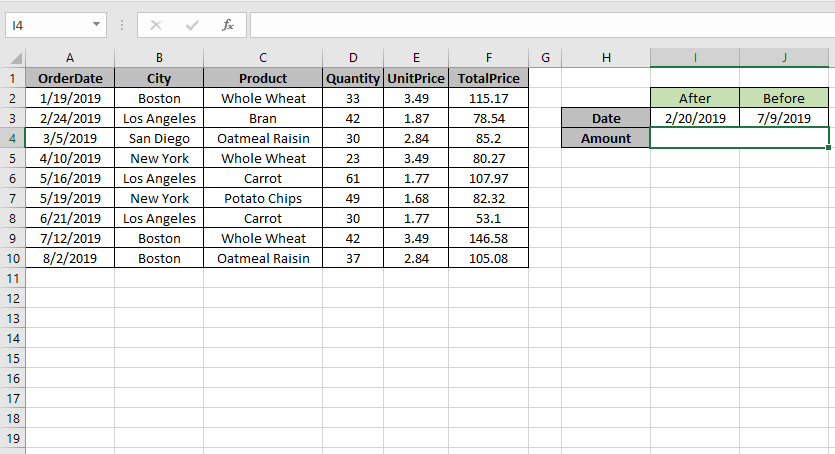Here we need to find the TotalPrice sum if date is between 2/20/2019 (after) & 7/9/2019 (before).

So we will use the formula to get the amount

 =SUMIFS (F2:F10 , A2:A10 , ">=" & I3, A2:A10 , "<=" & J3)

F2:F10 : Sum_range

A2:A10 : range where condition is applied

">=" & I3 : greater than date value in I3(2/20/2019).

"<=" & J3 : less than date value in J3.(7/9/2019).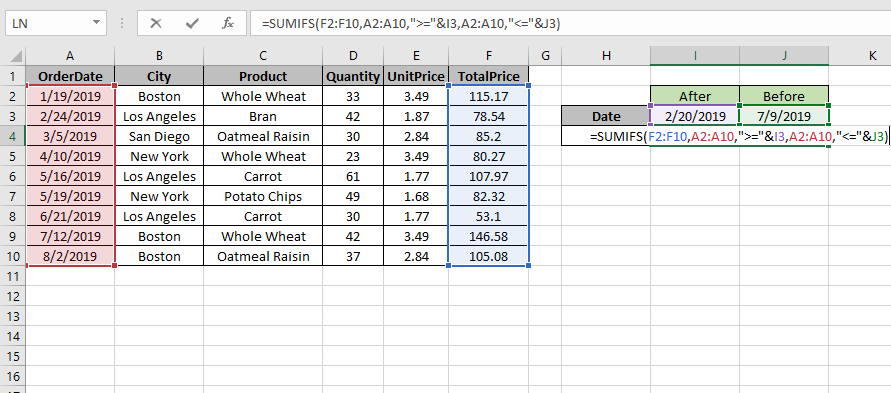Use the formula as stated above and click Enter.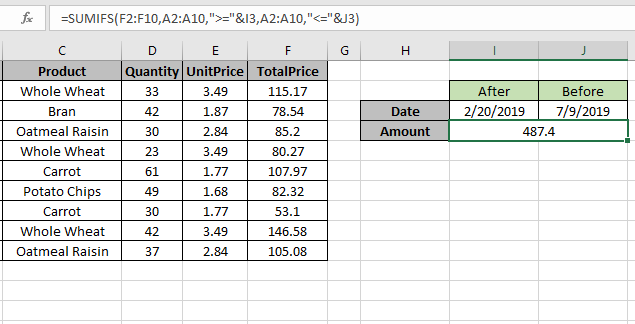As you can see the formula returns 487.4, the Totalprice between dates.

For customization just change the dates in I3 & J3 cell and get the results with the formula.

VBA Example :

All of these might be confusing to understand. Let's understand how to use the function using an example. Here We have Sales report for Salesman, Region, and Product for years 2012 to 2014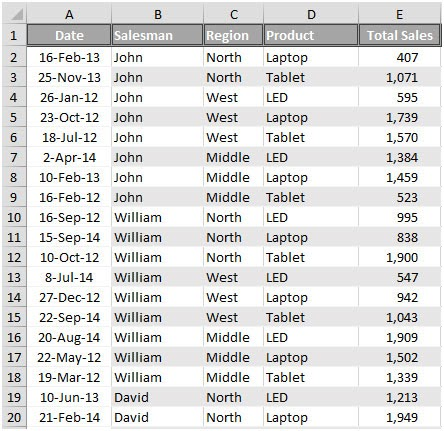We want to find out the Sales figure that meets the following conditions between 2 dates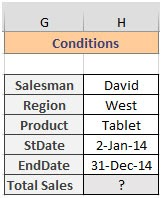To calculate Sales using VBA code, we have used OFFSET function to create Named ranges for each category.

Press CTRL + F3 to open Name Manager Window (the lists are already created)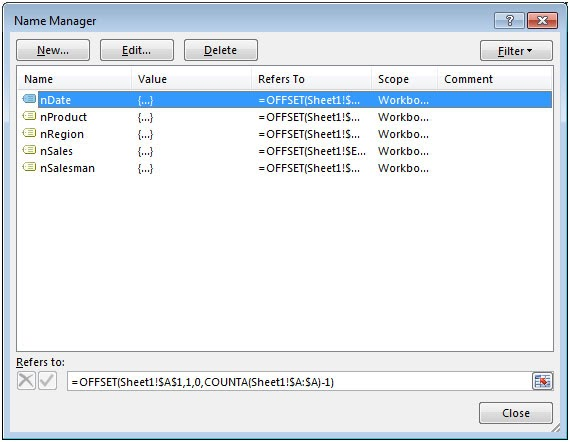Using Paste Names feature in Formulas tab, we will get the entire Defined Name range list in cells.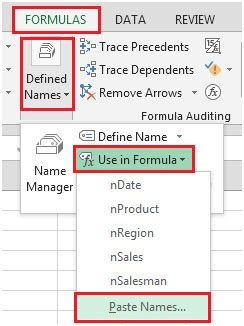Click on Paste List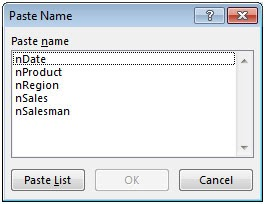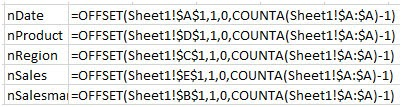We need to follow the below steps to launch VB editor

Click on Developer tab

From Code group, select Visual Basic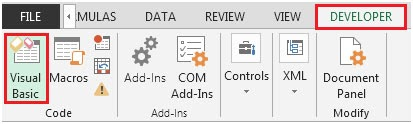Click on Insert, and then Module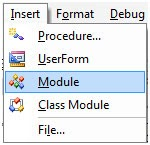This will create a new module.
Enter the following code in the Module

```Sub Sumifs2Dates()
mysalesman = [H3]
myregion = [H4]
myproduct = [H5]
stdate = [H6]
EndDate = [H7]

tsales = Application.WorksheetFunction.SumIfs([nsales], [nsalesman], mysalesman, [nregion], myregion, [nproduct], myproduct, [ndate], ">=" &stdate, [ndate], "<=" &EndDate)
[H8] = tsales
End Sub
```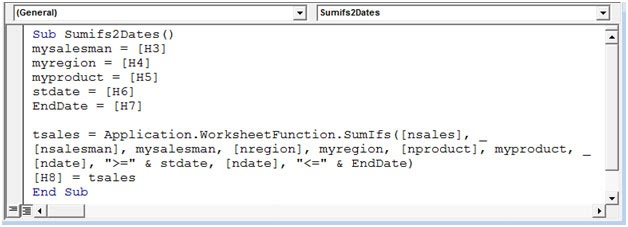Press ALT + F8 shortcut key for opening Macro window & then select the macro.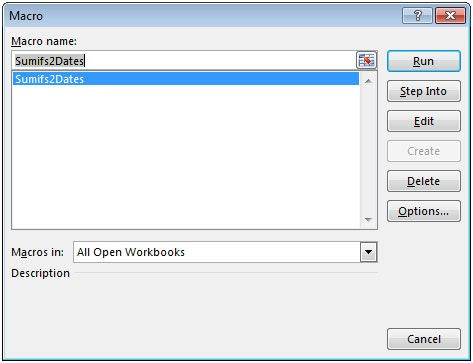Alternatively, you can press F5 to run the code in VBA screen.

After executing the macro, we will get the output in cell H8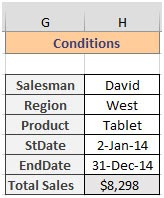This is how we can use SUMIFS function in VBA to calculate the total sales between 2 dates.

Here are all the observational notes using SUMIFs between Two Dates using function in Excel
Notes :

1. The formula only works with numbers.
2. The formula works only when there are no duplicates in the lookup table
3. The SUMPRODUCT function considers non - numeric values ( like text abc ) and error values ( like #NUM! , #NULL! )  as null values.
4. The SUMPRODUCT function considers logic value TRUE as 1 and False as 0.
5. The argument array must be of the same length else the function.

Hope this article about How to Use SUMIFs between Two Dates using VBA in Microsoft Excel is explanatory. Find more articles on SUMIF formulas and related Excel formulas here. If you liked our blogs, share it with your friends on Facebook. And also you can follow us on Twitter and Facebook. We would love to hear from you, do let us know how we can improve, complement or innovate our work and make it better for you. Write to us at info@exceltip.com.

Related Articles :

How to use the SUMPRODUCT function in Excel: Returns the SUM after multiplication of values in multiple arrays in excel.

SUM if date is between : Returns the SUM of values between given dates or period in excel.

Sum if date is greater than given date: Returns the SUM of values after the given date or period in excel.

2 Ways to Sum by Month in Excel: Returns the SUM of values within a given specific month in excel.

How to Sum Multiple Columns with Condition: Returns the SUM of values across multiple columns having condition in excel

How to use wildcards in excel : Count cells matching phrases using the wildcards in excel

Popular Articles :

How to use the IF Function in Excel : The IF statement in Excel checks the condition and returns a specific value if the condition is TRUE or returns another specific value if FALSE.

How to use the VLOOKUP Function in Excel : This is one of the most used and popular functions of excel that is used to lookup value from different ranges and sheets.

How to Use SUMIF Function in Excel : This is another dashboard essential function. This helps you sum up values on specific conditions.

How to use the COUNTIF Function in Excel : Count values with conditions using this amazing function. You don't need to filter your data to count specific values. Countif function is essential to prepare your dashboard.

Terms and Conditions of use

The applications/code on this site are distributed as is and without warranties or liability. In no event shall the owner of the copyrights, or the authors of the applications/code be liable for any loss of profit, any problems or any damage resulting from the use or evaluation of the applications/code.